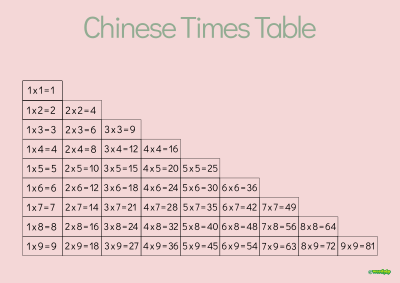# Chinese Multiplication Table

### worksheet number 118### This is a poster showing the multiplication table or times tables - as taught in China.

Times tables are taught in a slightly different way.

The tables are up to 9 times 9 ( 9 x 9 ) but omit any repeated calculations.
For example, there is no need to learn the 2 times table past 2 x 2 = 4

This is because you will also learn any other number past 2 x 2 in the other tables.

For example,
in the 3 times table there is 2 x 3 = 6
and in the 4 times times table there is 2 x 4 = 8

So repetition is avoided to try and keep it simple.

### Commutativity

Children are taught to recognize why 3 x 2 and 2 x 3 give the same result.
If a child can understand commutativity and have the memorised knowledge from their times tables that 3 x 2 = 6

they do not then need to have learned 2 x 3 = 6 as a separate, stand-alone piece of knowledge.

If you take the times tables and strip out the duplicates you get the essential elements of the times table### What this is for?

This is a poster showing the multiplication table or times tables as taught in China.
It is great for demonstrating commutativity.
Age range 5-8